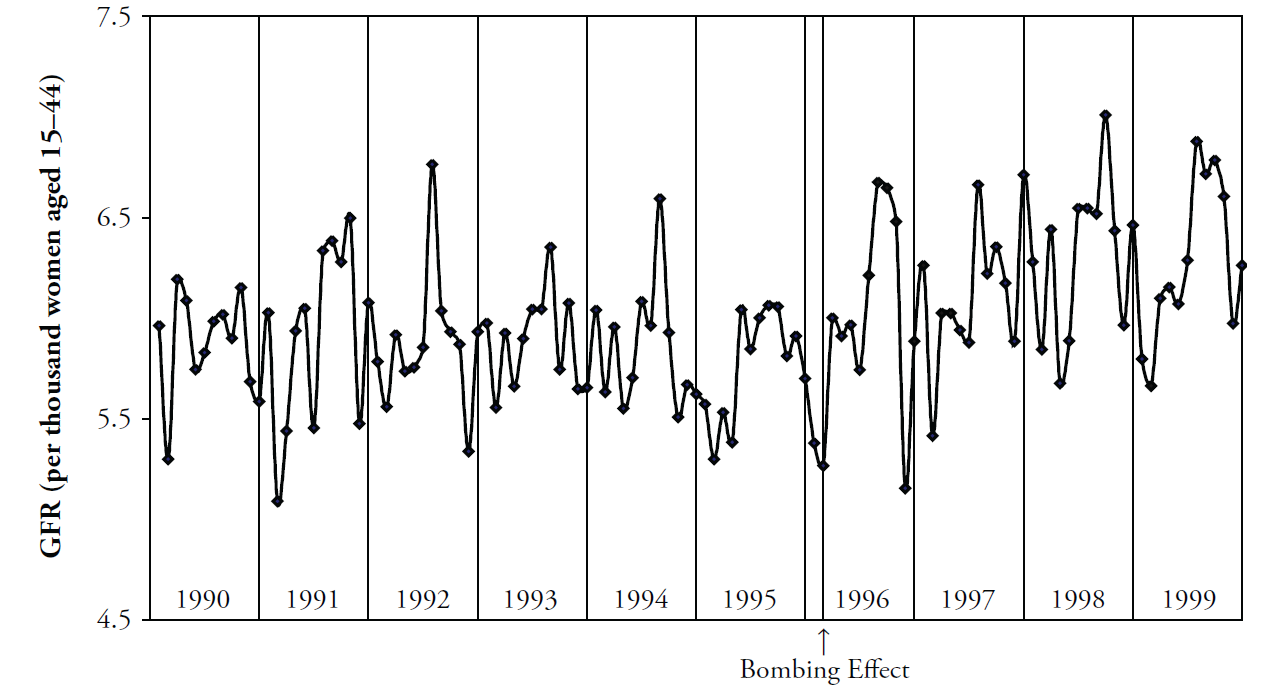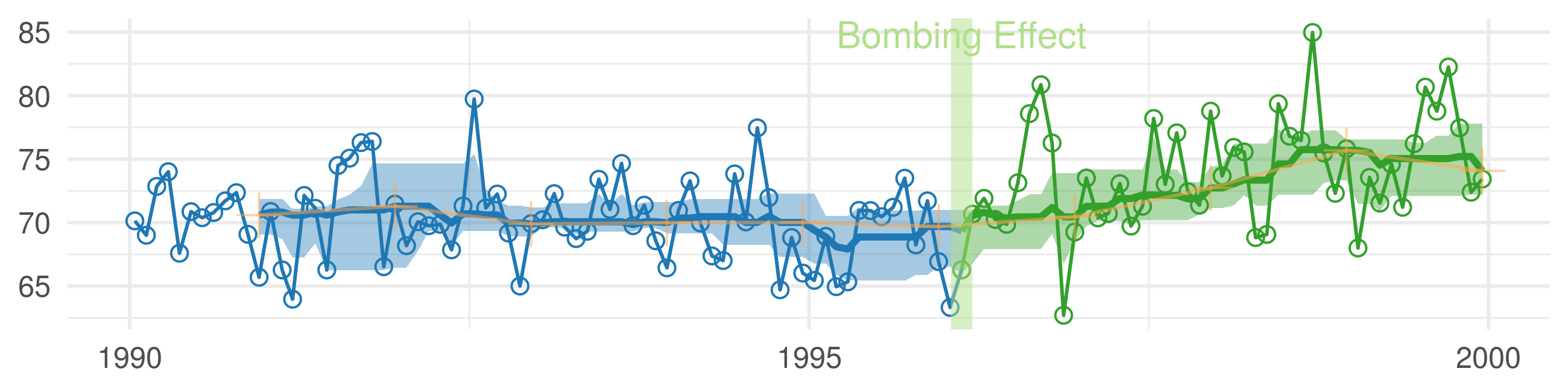# Figures for the MBR Manuscript

This vignette produces the graphs included in the initial MBR manuscript.

## Figure 1: Cartesian Rolling - 2005 Version

Figure 1: Raw monthly birth rates (General Fertility Rates; GFR's) for Oklahoma County, 1990-1999, plotted in a linear plot; the “bombing effect” is located ten months after the Oklahoma City bombing.## Figure 2: Cartesian Rolling - 2014 Version

Smoothed monthly birth rates (General Fertility Rates; GFRs) for Oklahoma County, 1990-1999, plotted in a linear plot. The top plot shows the connected raw data with a February smoother; the middle plot shows smoothing with a 12-month moving average, blue/green line, superimposed on a February smoother, red line); the bottom plot shows the smoothers and confidence bands, which are H-spreads defined using the distribution of GFR's for the given month and 11 previous months.

First, some R packages are loaded, and some variables and functions are defined.

``````changeMonth <- base::as.Date("1996-02-15") #as.Date("1995-04-19") + lubridate::weeks(39) = "1996-01-17"

vpLayout <- function(x, y) { grid::viewport(layout.pos.row=x, layout.pos.col=y) }

fullSpread <- function( scores ) {
return( base::range(scores) ) #A new function isn't necessary.  It's defined in order to be consistent.
}
hSpread <- function( scores ) {
return( stats::quantile(x=scores, probs=c(.25, .75)) )
}
seSpread <- function( scores ) {
return( base::mean(scores) + base::c(-1, 1) * stats::sd(scores) / base::sqrt(base::sum(!base::is.na(scores))) )
}
bootSpread <- function( scores, conf=.68 ) {
plugin <- function( d, i ) { base::mean(d[i]) }

distribution <- boot::boot(data=scores, plugin, R=99) #999 for the publication
ci <- boot::boot.ci(distribution, type = c("bca"), conf=conf)
return( ci\$bca[4:5] ) #The fourth & fifth elements correspond to the lower & upper bound.
}

darkTheme <- ggplot2::theme(
axis.title          = ggplot2::element_text(color="gray30", size=9),
axis.text.x         = ggplot2::element_text(color="gray30", hjust=0),
axis.text.y         = ggplot2::element_text(color="gray30"),
axis.ticks.length   = grid::unit(0, "cm"),
axis.ticks.margin   = grid::unit(.00001, "cm"),
#   panel.grid.minor.y  = element_line(color="gray95", size=.1),
#   panel.grid.major    = element_line(color="gray90", size=.1),
panel.margin        = grid::unit(c(0, 0, 0, 0), "cm"),
plot.margin         = grid::unit(c(0, 0, 0, 0), "cm")
)
``````
``````Warning: `axis.ticks.margin` is deprecated. Please set `margin` property of `axis.text` instead
``````
``````Warning: `panel.margin` is deprecated. Please use `panel.spacing` property instead
``````
``````lightTheme <- darkTheme + ggplot2::theme(
axis.title          = ggplot2::element_text(color="gray80", size=9),
axis.text.x         = ggplot2::element_text(color="gray80", hjust=0),
axis.text.y         = ggplot2::element_text(color="gray80"),
panel.grid.minor.y  = ggplot2::element_line(color="gray99", size=.1),
panel.grid.major    = ggplot2::element_line(color="gray95", size=.1)
)
dateSequence <- base::seq.Date(from=base::as.Date("1990-01-01"), to=base::as.Date("1999-01-01"), by="years")
xScale       <- ggplot2::scale_x_date(breaks=dateSequence, labels=scales::date_format("%Y"))
xScaleBlank  <- ggplot2::scale_x_date(breaks=dateSequence, labels=NULL) #This keeps things proportional down the three frames.
``````

### Individual Components

Here is the basic linear rolling graph. It doesn't require much specification, and will work with a wide range of approriate datasets. This first (unpublished) graph displays all components.

``````# dsLinearAll <- utils::read.csv("./Datasets/CountyMonthBirthRate2005Version.csv", stringsAsFactors=FALSE)
# dsLinearAll\$Date <- base::as.Date(dsLinearAll\$Date)
# dsLinearOkc <- dsLinearAll[dsLinearAll\$CountyName=="oklahoma", ]

# Uncomment the next two lines to use the version built into the package.  By default, it uses the
# CSV to promote reproducible research, since the CSV format is more open and accessible to more software.
dsLinearAll <- CountyMonthBirthRate2005Version
dsLinearOkc <- dsLinearAll[dsLinearAll\$CountyName=="oklahoma", ]

dsLinearOkc <- Wats::AugmentYearDataWithMonthResolution(dsLinear=dsLinearOkc, dateName="Date")

Wats::CartesianRolling(
dsLinear = portfolioCartesian\$dsLinear,
xName = "Date",
yName = "BirthRate",
stageIDName = "StageID",
changePoints = changeMonth,
changePointLabels = "Bombing Effect"
)
``````The version for the manuscript was tweaked to take advantage of certain features of the dataset. This is what it looks like when all three stylized panels are combined.

``````topPanel <- Wats::CartesianRolling(
dsLinear = portfolioCartesian\$dsLinear,
xName = "Date",
yName = "BirthRate",
stageIDName = "StageID",
changePoints = changeMonth,
yTitle = "General Fertility Rate",
changePointLabels = "Bombing Effect",
drawRollingBand = FALSE,
drawRollingLine = FALSE
)

middlePanel <- Wats::CartesianRolling(
dsLinear = portfolioCartesian\$dsLinear,
xName = "Date",
yName = "BirthRate",
stageIDName = "StageID",
changePoints = changeMonth,
yTitle = "General Fertility Rate",
changePointLabels = "",
drawRollingBand = FALSE,
drawJaggedLine = FALSE
)

bottomPanel <- Wats::CartesianRolling(
dsLinear = portfolioCartesian\$dsLinear,
xName = "Date",
yName = "BirthRate",
stageIDName = "StageID",
changePoints = changeMonth,
yTitle = "General Fertility Rate",
changePointLabels = "",
#   drawRollingBand = FALSE,
drawJaggedLine = FALSE
)

topPanel <- topPanel + xScale + darkTheme
middlePanel <- middlePanel + xScale + darkTheme
bottomPanel <- bottomPanel + xScaleBlank + darkTheme

grid::grid.newpage()
grid::pushViewport(grid::viewport(layout=grid::grid.layout(3,1)))
print(topPanel, vp=vpLayout(1, 1))
print(middlePanel, vp=vpLayout(2, 1))
print(bottomPanel, vp=vpLayout(3, 1))
grid::popViewport()
``````